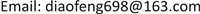1. 引言

2. 硬件在环半实物仿真平台

1) 列车制动距离试验值为733米，制动距离仿真值为748米，误差约为2%，满足对试验中制动距离模拟的相关要求；

2) 制动过程中任一时刻仿真车速与试验车速最大差值为1.06 km/h，满足要求；

3) 各轴轴速仿真值与试验值对比分析结果见表1，其中第3轴防滑判据较为特殊，不同于其他三根轴，在此不作分析。速度差均值统计误差最大为2.91%，标准差统计误差最大为4.81%，模型性能满足要求。

Statistical comparison between simulation values and experimental value
E ( △ v i )σ ( △ v i )

1轴3.893.880.262.702.574.81
2轴3.443.54−2.912.672.554.49
4轴3.353.291.792.532.433.95

3. 评价方法设计原则

1) 综合性原则

2) 全面性原则

3) 适应性原则

4) 强制性原则

5) 可重复性原则

4. 防滑控制系统性能评价方法建立

4.1. 防滑系统性能评价指标设计4.1.1. 黏着系数利用率指标

η = s min s r e a l × 100 % (1)

4.1.2. 耗风量增加比指标

V = ∫ Q d t (2)

k = V 滑 V 干 (3)

4.1.3. 轮对滑移做功指标

P = μ ⋅ T ⋅ Δ v (4)

W = ∫ μ ⋅ T ⋅ Δ v d t (5)

W a v g = ∑ 1 n W i n ( i = 1 , 2 , 3 , ⋯ , n ) (6)

4.1.4. 防滑阀动作次数指标

h a v g = ∑ 1 4 h i + ∑ 1 4 r j 8 (7)

4.2. 评价指标的归一化处理

x * = x − x min x max − x min , x * = 1 − x − x min x max − x min (8)

a 1 = η − 0 100 % − 0 (9)

a 2 = 1 − k − 1 25 − 1 (10)

a 3 = 1 − w − 0 26000 − 0 (11)

a 4 = 1 − h a v g − 0 300 − 0 (12)

4.3. 基于层次分析法的各黏着水平指标值权重确立

Judgement matrix scale and its meanin

1表示两个因素相比，具有同样重要性
3表示两个因素相比，一个因素比另一个因素稍微重要
5表示两个因素相比，一个因素比另一个因素明显重要
7表示两个因素相比，一个因素比另一个因素强烈重要
9表示两个因素相比，一个因素比另一个因素极端重要
2，4，6，8上述两相邻判断的中值

a i j = a i k / a j k ; i , j , k = 1 , 2 , 3 , ⋯ , n

1) 计算判断矩阵每一行元素的积，其中 n 为矩阵阶数：

M i = ∏ j = 1 n b i j , i = 1 , 2 , 3 ⋯ n (13)

2) 计算各行的 n 次方根值： w ¯ i = M i n , i = 1 , 2 , 3... ⋯ n ；

3) 将向量 ( M ¯ 1 , M ¯ 2 , M ¯ 3 , ⋯ . M ¯ n ) T 归一化，计算如下，即为所求各指标的权重系数：

w i = w ¯ i ∑ j = 1 n w ¯ j (14)

4) 判断矩阵的最大特征根为：

λ max = ∑ i = 1 n ( A W ) i n w i (15)

Mean random consistency index R
n12345678910111213
RI000.520.891.121.261.361.411.461.491.521.541.56

5. 结束语

1) 基于综合性、全面性、适应性、强制性、可重复性等原则，从列车制动全过程黏着系数利用率、耗风量扩大比、轮对滑移做功、防滑阀动作次数等四个角度建立列车防滑系统评价方法；

2) 从多种轮轨黏着水平下对其性能进行考虑，使用min-max离差标准化及层次分析法对各指标进行归一化和标准化处理；

3) 确立了各性能指标所需采集量、计算方法以及数据归一化过程中各参数值的选取；

4) 完成层次分析法判断矩阵的建立及各指标权重的计算和一致性校验。